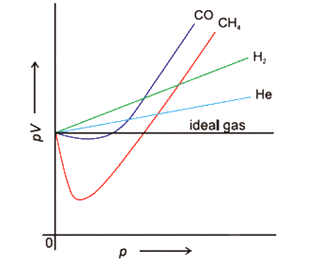# Mathematical equation for the deviation from ideality of real gases

phantomvommandHi guys,

We have this very common graph where pV deviates from ideality.

May I know the equation for such a curve?

Secondly, if the x-axis were changed to V, what would the graph look like?

Homework Helper
Check out equation of state
or consult a book like e.g. P.W. Atkins: physical chemistry

##\ ##

•vanhees71 and phantomvommand
Mentor
View attachment 290237
Hi guys,

We have this very common graph where pV deviates from ideality.

May I know the equation for such a curve?

Secondly, if the x-axis were changed to V, what would the graph look like?
The vertical axis is labelled incorrectly. It should read $$\frac{PV}{RT}$$where V is the molar volume. This is called the compressibility factor Z. According to the Principle of Corresponding States, Z is a function of the reduced pressure ##P/P_C##, the reduced temperature ##T/T_C##, and the ascentric factor ##\omega##, where the subscript C indicates the value at the critical point and where ##\omega## varies with the specific gas. Graphs of Z as a function of reduced temperature, reduced pressure, and ascentric factor can be found in most thermo books.

•BvU and vanhees71